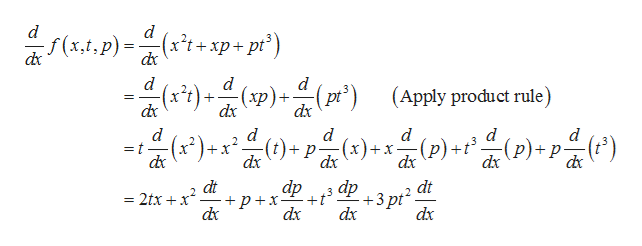# dversusdxOx

Question
6 views

Whats the difference?

check_circle

Step 1

What is the difference between?

Step 2

The normal derivative assumes each variable as a function where the partial derivative assumes x variable as a function and other variables as constant.

Step 3

The normal deriv...help_outlineImage Transcriptionclosed d (x't+xp+ pt') -f(x,t,p) ()p)) d d d (x't)- (pt2 (Apply product rule) -(хр). dx + dx d d d d d (x2 dx (p)+tp)p -(x) dx x dx dx dx dp dp t dx dx dt p+x d dt 2 +3pt dx 2txx = fullscreen

### Want to see the full answer?

See Solution

#### Want to see this answer and more?

Solutions are written by subject experts who are available 24/7. Questions are typically answered within 1 hour.*

See Solution
*Response times may vary by subject and question.
Tagged in

### Math